American Journal of Optics and Photonics
Volume 3, Issue 2, April 2015, Pages: 30-33

Reflection and Transmission of Light Beams at a Curved Interface: Coherent State Approach

Nikolai I. Petrov

Scientific and Technological Centre of Unique Instrumentation, Russian Academy of Sciences, Moscow, Russia

Email address: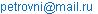To cite this article:

Nikolai I. Petrov. Reflection and Transmission of Light Beams at a Curved Interface: Coherent State Approach. American Journal of Optics and Photonics. Vol. 3, No. 2, 2015, pp. 30-33. doi: 10.11648/j.ajop.20150302.12

Abstract: Phase-space procedure based on coherent state representation is proposed for investigation of reflection and transmission of wave beams at a curved dielectric boundary. Numerical simulations of reflection and transmission of light at various boundaries separating two different dielectrics are carried out. Significant influence of wave-front curvature and polarization of incident beam on the reflectance and transmittance is shown.

Keywords: Reflection and Transmission, Coherent States, TE and TM Polarized Beams

Contents

1. Introduction

Micro-optical elements are widely used in modern optical systems, such as light homogenizers, micro-lens arrays, solar cells, etc. For consideration of large aperture micro-optical systems, conventional methods, such as physical optics, become overly time-consuming. Beam-mode representations do not provide right alternative, since the beam modes cannot be tracked simply through the arbitrarily curved surfaces. Discrete phase-space methods [1,2] have been proposed as an efficient alternative. These methods represent fields as discrete and finite superposition of elementary Gaussian beams that can be traced easily in a complicated environment. However, the method usually proposed to discrete fields, the so-called Gabor representation, has been shown numerically unstable [3,4]. In  the concept of field tracing is introduced that is the generalization of ray tracing and enables electromagnetic system modelling.

In this Letter, the approach based on coherent state representation is proposed for analysis of reflection and transmission of light beam at a curved dielectric surface profile.

Coherent states are used in many different areas of physics [6,7]. In  the coherent state method was used for consideration of nonparaxial propagation and focusing of wave beams in a graded-index medium. The term "coherent states" was introduced by Glauber  for a one-dimensional steady-state quantum oscillator in connection with problems in quantum optics. Such states were constructed and investigated already by Schrodinger in 1926  in order to establish a relationship between the classical and quantum approaches.

2. Formulation of the Problem

Consider the curved boundary between two different dielectric media (Fig. 1). The absence of media losses is assumed below. For simplicity, the two-dimensional periodically corrugated interface (y–independent) with the period d >> l is considered, but the extension to the arbitrary profile of 3D case is straightforward.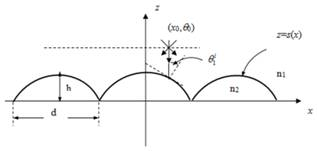Fig. 1. Geometrical configuration and coordinate system for a boundary.

The calculation procedure of the reflected and transmitted powers consists of the following steps. At first, the incident beam field is expanded into coherent states, representing elementary Gaussian beams with axis displacement and tilt. Note, that in a sense such Gaussian beams are similar to the complex rays used for simulation of reflection and transmission of beams at a curved interface in . Coherent states (CS) form a full set of functions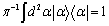, so the arbitrary incident field E(x,0) can be expanded into a set of CS: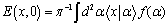(1)

where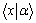is given by the expression (3),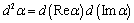is the elementary phase-space volume,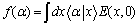are the amplitudes of the expansion.

Coherent state can be determined as the eigenfunction of the annihilation operator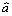: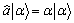(2)

where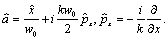An explicit form of CS is given by a Gaussian beam function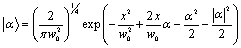(3)

where the complex eigenvalues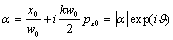determine the initial coordinates x0 of the center of the elementary beam and the angle of its inclination px0 = n0sinq0 to the z-axis, n0 is the refractive index of medium, w0 is the elementary beam width, k = 2p/l is the wavenumber.

Note, in contrast to Fourier-expansion, there is no requirement for orthogonality of functions, owing to CS form overfull function system. The square of the modules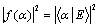determine the incident beam power distribution between the elementary beams (CS). For the incident Gaussian beam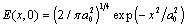the amplitudes of expansion have the form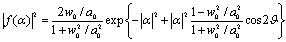(4)

The Gaussian elementary beams (CS) pass through the interface as determined by the corresponding Fresnel coefficients that vary according to the angle of incidence. Usually the Fresnel formulae are known from the plane–wave limit. However, these formulae can be used also for localized wave beams with the beam waists w > l [11, 12].

Finally, total reflected and transmitted powers are determined by a summation of powers of all elementary beams. The reflectance and transmittance are defined as the ratios of reflected and transmitted powers to the incident power, accordingly: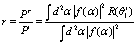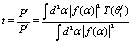(5)

where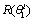and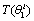are the reflection and transmission coefficients,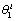is the incident angle.

The reflection and transmission coefficients for TE (transverse electric) and TM (transverse magnetic) linearly polarized incident beams, accordingly, are given by the expressions :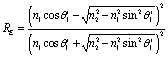(6)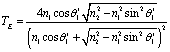where n1 and n2 are the refractive indexes of media 1 and 2, accordingly.

3. Simulation Results

For the surface-relief profile z=s(x), the ray (CS) with initial coordinates (x0,q0) strikes the interface at the point (x1, z1), where the corresponding incidence angleis uniquely determined. For example, the incident angle can be expressed as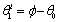, where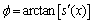,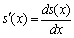is the derivative of the surface profile function with respect to the x coordinate.

Results of simulation are presented for different surface profiles, wavefront curvature radiuses and polarizations of incident beam. The parameters for incident beam, surface-relief, and elementary beam are in the ratio a0 > d >> w0 > l, where d is the diameter of the single element in corrugated surface (Fig.1). Fig. 2 shows the reflectance and transmittance as function of sag h of the surface relief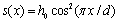with the period d = 50mm for TE and TM polarized beams with different wavefront curvature radiuses. Analogical dependences are obtained for the parabolic surface profile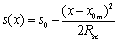, where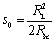, RL = d/2 and Rsc are the radius and curvature radius of the single element, x0m is the center coordinate of the single element (Fig. 3). Lesser sensitivity of the reflection and transmission to the sag h and wavefront curvature radius Rf changes is observed for parabolic surface profile. Reflectance increases and transmittance decreases with the increase of sag h. The reflectance and transmittance are sensitive to the wavefront curvature radius and polarization of the incident beam. For lower-higher index interface the reflectance is lower and transmittance is higher for TM polarized beam if h < d. For higher-lower index interface there is no evident difference between TE and TM polarizations. It follows from the simulations that the decrease of the reflectance and increase of the transmittance take place with the increase of the sag h for TM polarization owing to Brewster angle effect.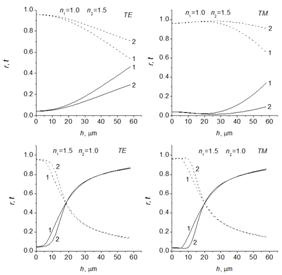Fig. 2. Reflectance r (solid curves) and transmittance t (dashed curves) versus depth h at lower/higher index and higher/lower index interfaces for different values of incident wavefront curvature radiuses: left - TE polarized beam, right – TM polarized beam; curves 1, Rf = 1500 mm; curves 2, plane wavefront.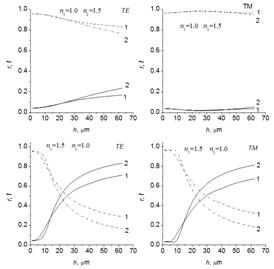Fig. 3. Reflectance r (solid curves) and transmittance t (dashed curves) versus depth h at lower/higher index and higher/lower index interfaces with the parabolic profile for different values of incident wavefront curvature radiuses: left - TE polarized beam, right – TM polarized beam; curves 1, Rf = 1500 mm; curves 2, plane wavefront.

The proposed method is an efficient alternative to model aperture functions and flat-topped laser beams. Unlike the superposition of off-axis Gaussian function components used in , CS decomposition represents the superposition of linearly shifted and spatially rotated beams, forming the full set of functions. Transfer-matrix method  can be used to calculate the propagation of off-axis Gaussian beams in optical systems with tilted, displaced and curved optical elements.

4. Conclusion

The CS approach can be used for simulation of intensity distribution of light diffracted by the micro-lens array [16, 17]. As illustrated by simulations, the optical efficiency (transmittance) of such systems strongly depends on the aspect ratio h/d. For h/d <1 the transmittance of micro-lens arrays exceeds 90%, which is in good agreement with the existing experimental data. The proposed method is an efficient alternative to model aperture functions and flat-topped laser beams.

Thus, the CS based continuous decomposition has been presented for simulation of light beam reflection and transmission at arbitrarily curved surfaces. Reflectance and transmittance of light beam at different surface-reliefs with depths h >> l are investigated. This method provides effective algorithm to model complicated structures (dielectric antennas, lenses, multiple interfaces, solar cells, etc.) with the help of combination of wave propagation and ray-tracing procedures.

References

1. P.D. Einziger, S. Raz, and M. Shapira, "Gabor representation and aperture theory", J. Opt. Soc. Am. A, 1986, 3, pp. 508-522.
2. B.Z. Steinberg, E. Heyman, and L.B. Felsen, "Phase-space beam summation for time-harmonic radiation from large apertures", J. Opt. Soc. Am. A, 1991, 8, pp. 41-59.
3. I. Daubechies, "The wavelet transform, time-frequency localization and signal analysis", IEEE Trans., 1990, IT-36, pp. 961-1005.
4. D. Lugara, and C. Letrau, "Alternative to Gabor’s representation of plane aperture radiation", Electronics Letters, 1998, 34, pp.2286-2287.
5. F. Wyrowski, and M. Kuhn, Introduction to field tracing, J. Mod. Opt., 2011, 58, 449-466.
6. R.J. Glauber, "Coherent and incoherent states of the radiation field", Phys. Rev., 1963, 131, pp. 2766-2788.
7. J.R. Klauder and B.S. Skagerstam, Coherent states, Singapore: World Scientific, 1985.
8. N.I. Petrov, "Focusing of beams into subwavelength area in an inhomogeneous medium", Optics Express, 2001, 9, pp. 658-673.
9. E.S. Schrodinger, "Der Stetige Ubergang von den Mikro- zur Makromechanik," Naturwissenschaften, 1926, 14, pp. 664-666.
10. Y.Z. Ruan, and L.B. Felsen, "Reflection and transmission of beams at a curved interface," J. Opt. Soc. Am. A, 1986, 3, 566-579.
11. N.I. Petrov, "Reflection and transmission of strongly focused vector beams at a dielectric interface", Optics Letters, 2004, 29, pp. 421-423.
12. N.I. Petrov, "Reflection and transmission of strongly focused light beams at a dielectric interface", J. Mod. Opt., 2005, 52, no. 11, pp. 1545-1556.
13. D. Marcuse, Light Transmission Optics, Chapter I, New York, 1972.
14. A.A. Tovar, Propagation of flat-topped multi-Gaussian laser beams, J. Opt. Soc. Am. A, 2001, 18, 1897-1904.
15. A.A. Tovar, and L.W. Casperson, "Generalized beam matrices: Gaussian beam propagation in misaligned complex optical systems," J. Opt. Soc. Am. A, 1995, 12, 1522-1533.
16. N. Lindlein, "Simulation of micro-optical systems including microlens arrays," J. Opt. A: Pure Appl. Opt., 2002, 4, S1-S9.
17. H. Urey, and K.D. Powell, "Microlens-array-based exit-pupil expander for full-color displays," Applied Optics, 2005, 44, 4930-4936.

 Contents 1. 2. 3. 4.
Article ToolsAbstractPDF(218K)
Follow on us
PUBLICATION SERVICE
JOIN US
RESOURCES
SPECIAL SERVICES
ADDRESS
Science Publishing Group
548 FASHION AVENUE
NEW YORK, NY 10018
U.S.A.
Tel: (001)347-688-8931